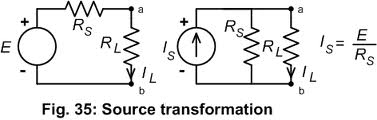## Source Transformations Assignment Help

Assignment Help: >> Dc Circuits - Source Transformations

Source Transformations:

The voltage and current sources are mutually transferrable. Any practical voltage source (or simply, a voltage source) contain an ideal voltage source in series with an internal resistance (for ideal source, this impedance is being zero and the output becomes independent of the load current).

Any practical current source is demonstrated by ideal current source in parallel with their internal resistance. Let Figures (a) and (b) demonstrates their equivalence.(a) A Practical Voltage Source           (b) A Practical Current Source

Figure: Source Transformation

Suppose some load resistance rL connected at terminal a-b of both voltage and current source.

I La   = V / r a  + r L

I Lb        = I (rb / rb  + rL)

Two sources to become identical they must deliver same current to load

V/ ra  + rL       = I (rb /rb  + rL)

Though, for the current source, the terminal voltage at a-b would be I rb, a-b being open

i.e.  V = I rb

Now, finally we get

i.e.  ra  + rL  = rb  + rL

ra  = rb

Thus, for any practical voltage source, if ideal voltage is V and internal resistance is ra, the voltage source, may be replaced by a current source I with the internal resistance in parallel to the current source.